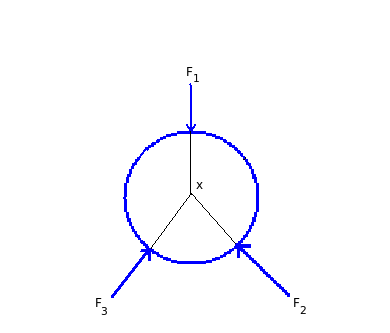# Concurrent forces - forces passing through a common point

Q.  Forces passing through a common point are known as _____
- Published on 18 Sep 15

a. collinear forces
b. co-planer forces
c. concurrent forces
d. none of the above

#### Discussion

• Sravanthi   -Posted on 14 Dec 15
- Forces passing through a common point are known as concurrent forces.- Forces F1, F2, F3 pass through a common point X.

- If any one force does not pass through the common point, then it creates an unbalanced moment which causes rotation.

- Therefore, for a body to be equilibrium state not only the forces should pass through a common point but also they should have same magnitude and direction.

- The condition, Σ Fx = Σ Fy = 0 should be satisfied.

- Tug of war is a good example for concurrent forces. The concurrent forces intersect at a same point i.e at the middle of rope.

## ➨ Post your comment / Share knowledgeEnter the code shown above:

(Note: If you cannot read the numbers in the above image, reload the page to generate a new one.)